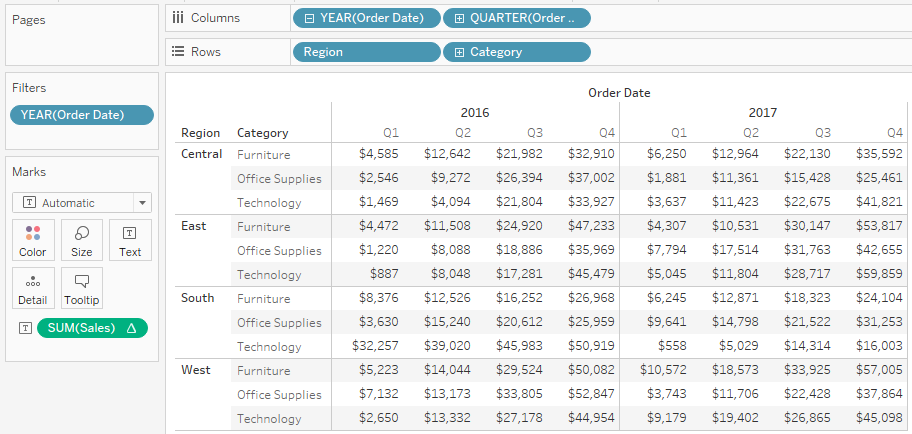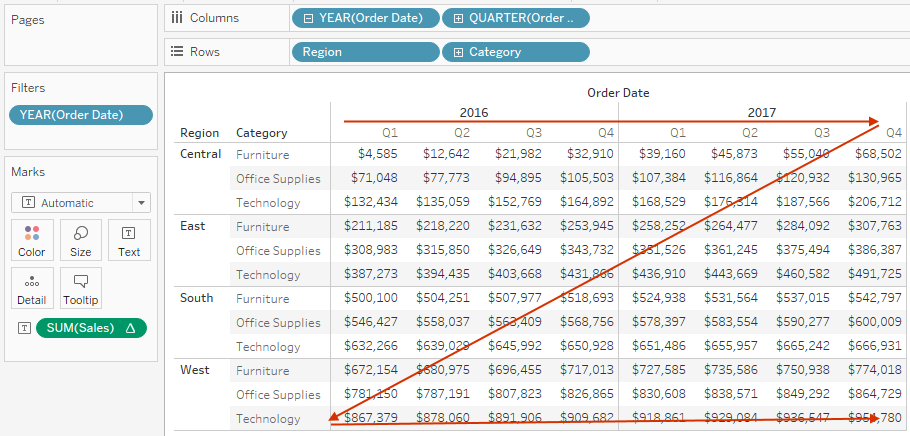top of page
Search
• Bernard K

# Table Calculations in Tableau: Running TotalRunning total is one of most used table calculation in Tableau. It is used to calculate the cumulative total of a measure across a table structure or specific dimension. It can do this by summing values, averaging values or by replacing all values by the lowest or highest value etc.

To create a running total calculation you need to know the following;

• Type of aggregation to use in summarizing the values e.g. MAX, MIN, AVG, etc

• The dimension or table structure (table, pane, cell) along which the calculation will be done. Note: the default calculation is set to be “Table (Across)

The following is an example of running table window;(This is the default setting where the calculation is set to be computed ‘across the table’)

Resulting to this table with values accumulated across the table.More configurations such as computing using a specific dimension or restarting the computation every year can be set as follows;Giving us a different view where computation is restarted every year.Other options include;

## Computing Table (across then down)## Computing Table (down then across)More and more options can be revealed under the running total dialogue box

If this was helpful to you, kindly subscribe to our mailing list to stay tuned anytime we publish